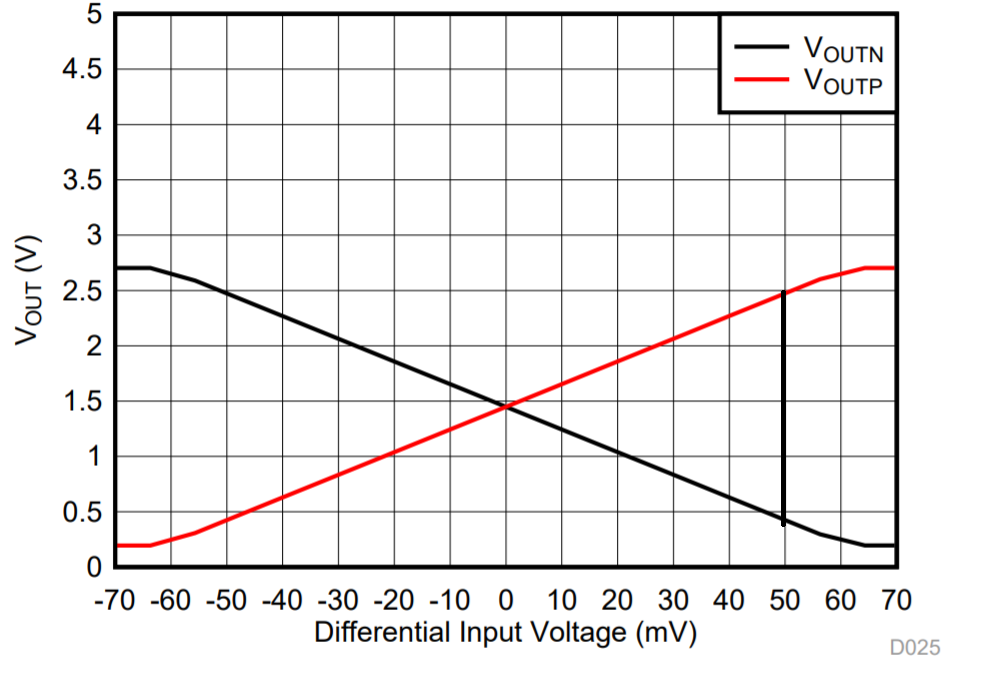If you have a related question, please click the "Ask a related question" button in the top right corner. The newly created question will be automatically linked to this question.

AMC1302-Q1: Peak to peak output voltage of the AMC1302-Q1

Part Number: AMC1302-Q1

Hi,

In fact, I have read about Automotive shunt-based isolated current sensor reference design ( http://www.ti.com/lit/ug/tiduel5/tiduel5.pdf?ts=1588591688374) in the section 2.4.2  that  this 50-mV device is amplified at a gain factor of 41, which results in output voltage levels of -2.05 V to +2.05 V on the differential output stage of an isolated amplifier. This means that it amounts to a peak-to-peak voltage of 4.10 V on the output terminals of the AMC1302-Q1, but when we open the datasheet of the AMC1302-Q1 we see in figure 26 that the differential output is approximately 2.05V when the differential input is 50mV. My question is why did they say that the output terminal peak to peak is 4.10V when it should be 2.05 V based on the figure that I joined and thank you in advance.• Hello Hicham,

The gain of a fully differential amplifier is referring the differential output (VOUTP - VOUTN) to the differential input (VINP - VINN). As you have already done based on this plot, if you take the point of +50 mV input and calculate the differential output, you will get a result of +2.05 V (roughly 2.45 V - 0.4 V). Similarly if you take the point of -50 mV input and calculate the differential output, you will get -2.05 V (roughly 0.4 V - 2.45 V). Here you can see the full +/- 2.05 V range which is described in the datasheet.

Regards,